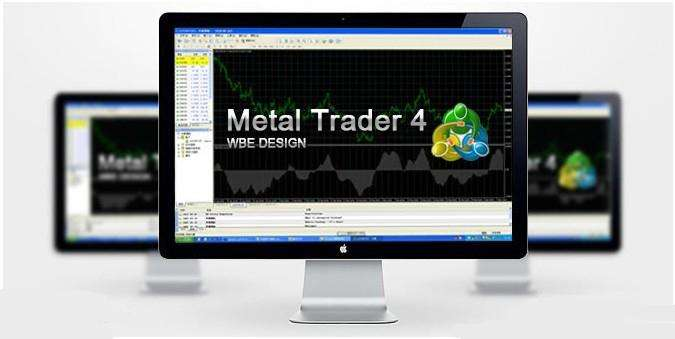# mt4快速交易技巧（附MT4常用快捷键大全）

## 2022-08-10    分类:外汇    阅读:450MT4最全快捷键分类

Ctrl系列

Ctrl+M=市场报价

Ctrl+N=导航

Ctrl+I=打开指标窗口;

Ctrl+S=保存选择的图表形式

Ctrl+P=用黑白颜色打印图表;

Ctrl+T=帐号终端窗口

Ctrl+R=打开/关闭"测试"窗口

Ctrl+D=数据窗口

Ctrl+G=网格

Ctrl+L=成交量

Ctrl+O=选项

Ctrl+F=十字光标

Ctrl+Y=在图表上显示日期线

Ctrl+W=关闭当前图表窗口

Ctrl+Z or Alt+Backspac=取消删除对象

F系列

F1=获得帮助

F2=历史数据中心

F3=全局变量

F4=MT程序编辑器MetaEditor

F5=图标窗口重绘

F6=交易系统测试

F7=交易系统参数设置

F8=图表主窗口属性

F9=订单

F10=弹出报价

F11=图标窗口全屏

F12=步进的方式向左移动图表

+=图表放大

-=图表缩小

←=图表向左移动

↑=图表向左移动

→=图表向右移动

↓=图表向右移动

Home—移动图表到开始;

End—移动图表到最后

Alt+1=美国线

Alt+2=蜡烛图

Alt+3=折线图

Alt+W—显示图表控制窗口

Shift+F12=步进的方式向右移动图表

Shift+F5=显示前一个数据图表

4个常用的交易快捷方式

1、快速开仓

2、快速平仓

3、快速设止损止盈

4、回溯交易历史

MT4快捷键，一个细节，彰显你操盘手的交易素养。